# Summary - Theoretical Distribution Notes | Study Business Mathematics and Logical Reasoning & Statistics - CA Foundation

## CA Foundation: Summary - Theoretical Distribution Notes | Study Business Mathematics and Logical Reasoning & Statistics - CA Foundation

The document Summary - Theoretical Distribution Notes | Study Business Mathematics and Logical Reasoning & Statistics - CA Foundation is a part of the CA Foundation Course Business Mathematics and Logical Reasoning & Statistics.
All you need of CA Foundation at this link: CA Foundation

SUMMARY

1. A probability distribution also possesses all the characteristics of an observed distribution. We define population mean (μ) , population median (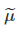) , population mode (μ0 ) , population standard deviation (σ) etc. exactly same way we have done earlier. These characteristics are known as population parameters.
2. Probability distribution or a Continuous probability distribution depending on the random variable under study.
3. Two important discrete probability distributions are (a) Binomial Distribution and (b) Poisson distribution.
4. Normal Distribution is a important continuous probability distribution
5. A discrete random variable x is defined to follow binomial distribution with parameters n and p, to be denoted by x ~ B (n, p), if the probability mass function of x is given by
f (x) = p (X = x) = ncx px.qn-x  for x = 0, 1, 2, …., n
= 0, otherwise
6. Additive property of binomial distribution. If X and Y are two independent variables such that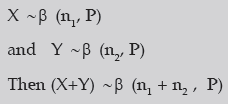7. Definition of Poisson Distribution
A random variable X is defined to follow Poisson distribution with parameter λ, to be denoted by X ~ P (m) if the probability mass function of x is given by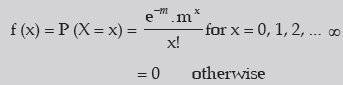1. Since e–m = 1/em >0, whatever may be the value of m, m > 0, it follows that f (x) ≥ 0 for every x.
Also it can be established that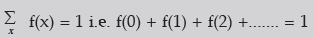2. Poisson distribution is known as a uniparametric distribution as it is characterised by only one parameter m.
3. The mean of Poisson distribution is given by m i.e μ = m.
4. The variance of Poisson distribution is given by σ2 = m
5. Like binomial distribution, Poisson distribution could be also unimodal or bimodal depending upon the value of the parameter m.
6. Poisson approximation to Binomial distribution
7. Additive property of Poisson distribution
8. A continuous random variable x is defined to follow normal distribution with parameters μ and σ2, to be denoted by X ~ N (μ,σ2 )
If the probability density function of the random variable x is given by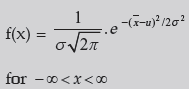where μ and σ are constants, and σ > 0
9. Properties of Normal Distribution
• Since π = 22/7 , e–θ= 1/eθ > 0, whatever θ may be, it follows that f (x) ≥ 0 for every x.
It can be shown that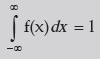• The mean of the normal distribution is given by μ. Further, since the distribution is symmetrical about x = μ, it follows that the mean, median and mode of a normal distribution coincide, all being equal to μ.
• The standard deviation of the normal distribution is given by σ Mean deviation of normal distribution is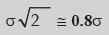The first and third quartiles are given by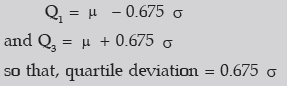• The normal distribution is symmetrical about x = μ . As such, its skewness is zero i.e. the normal curve is neither inclined move towards the right (negatively skewed) nor towards the left (positively skewed).
• The normal curve y = f (x) has two points of inflexion to be given by x = μ – σ and x = μ + σ i.e. at these two points, the normal curve changes its curvature from concave to convex and from convex to concave.
• if x ~ N ( μ , σ2 ) then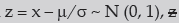is known as standardised normal variate or normal deviate.
We also have P (z ≤ k ) = ϕ (k)
• Area under the normal curve is shown in the following figure: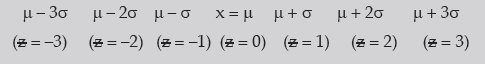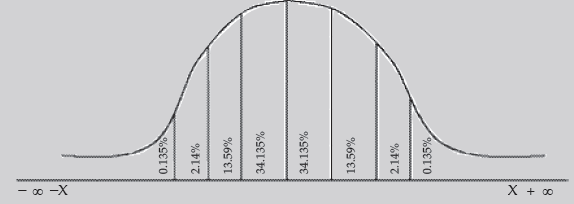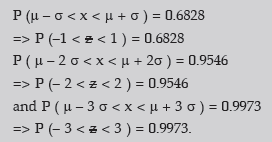• We note that 99.73 per cent of the values of a normal variable lies between (μ – 3 σ) and (μ + 3 σ). Thus the probability that a value of x lies outside that limit is as low as 0.0027.
• If x and y are independent normal variables with means and standard deviations as μ1 and μ2 and σ1, and σ2 respectively, then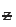= x + y also follows normal distribution with mean (μ1 + μ2) and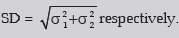• Standard Normal Distribution
If a continuous random variablefollows standard normal distribution, to be denoted by~ N(0, 1), then the probability density function of z is given by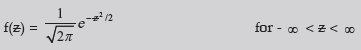Some important properties of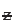are listed below:
(i)has mean, median and mode all equal to zero.
(ii) The standard deviation ofis 1. Also the approximate values of mean deviation and quartile deviation are 0.8 and 0.675 respectively.
(iii) The standard normal distribution is symmetrical about= 0.
(iv) The two points of inflexion of the probability curve of the standard normal distribution are –1 and 1.
(v) The two tails of the standard normal curve never touch the horizontal axis.
(vi) The upper and lower p per cent points of the standard normal variable z are given by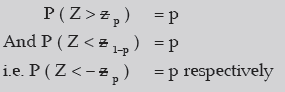(since for a standard normal distribution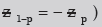Selecting P = 0.005, 0.025, 0.01 and 0.05 respectively,

We have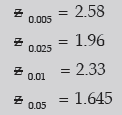These are shown in fig 17.3.
(vii) If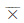denotes the arithmetic mean of a random sample of size n drawn from a normal population then,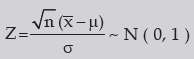The document Summary - Theoretical Distribution Notes | Study Business Mathematics and Logical Reasoning & Statistics - CA Foundation is a part of the CA Foundation Course Business Mathematics and Logical Reasoning & Statistics.
All you need of CA Foundation at this link: CA FoundationUse Code STAYHOME200 and get INR 200 additional OFF

## Business Mathematics and Logical Reasoning & Statistics

81 videos|101 docs|87 tests

Track your progress, build streaks, highlight & save important lessons and more!

,

,

,

,

,

,

,

,

,

,

,

,

,

,

,

,

,

,

,

,

,

;